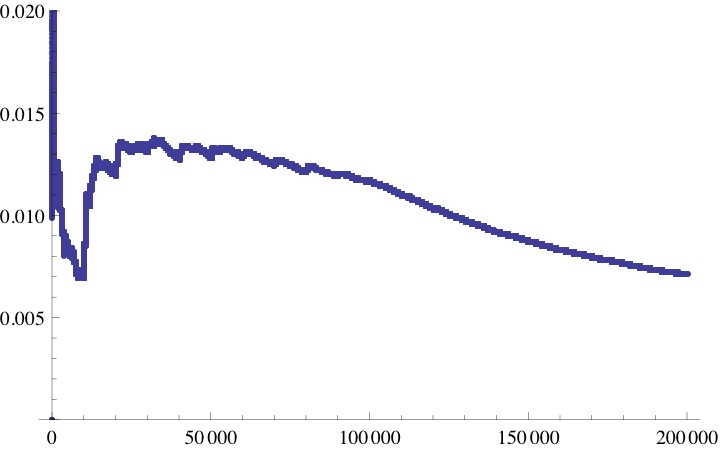# A curious number exploration

Posted by: Gary Ernest Davis on: May 6, 2011

Benjamin Vitale (@BenVitale) tweeted that he followed 203 people on Twitter and$203^2 + 203^0 + 203^3 = 8406637$ is a prime number.

Alex Bogomolny (@CutTheKnotMath) observed that if$n=abc$ (digits base 10) then$abc^a+abc^b+abc^c$ has a factor of$abc$ unless one of$a, b, c =0$.

A quick computational search (using Mathematicaâ„¢) yields the following numbers$n=d_k\ldots d_0 \leq 10000$ (base 10 digits) for which$n^{d_k}+\ldots +n^{d_0}$ is prime:

10, 20, 203, 230, 308, 309, 330, 350, 503, 603, 650, 960, 1068, 1110, 1206, 1350, 1404, 1480, 1730, 1802, 1860, 1910, 2032, 2038, 2044, 2054, 2250, 2320, 2502, 3044, 3082, 3402, 3970, 4032, 4046, 4072, 4120, 4340, 4450, 4540, 4650, 4908, 5204, 5310, 5402, 5530, 5770, 5820, 6038, 6076, 6120, 6206, 6490, 6520, 6830, 7110, 8074, 8106, 8120, 8380, 8450, 8610, 8690, 8902, 8960, 9064, 9330, 9402, 9408, 9950

How do these numbers increase?

Are they becoming relatively rarer?

Let’s denote by$V(p)$ the number of$n=d_k \ldots d_0 \textrm{ (base 10 digits) } \leq p$ such that$n^{d_k}+\ldots + n^{d_0}$Â  is prime.

How does the fraction$\frac{V (p)}{p}$ vary with increasing$p$?

Here is a plot of that fraction up to$p=200000$:It appears that$\frac{V(p)}{p} \to 0$ as$p$ increases, although the decrease is highly exaggerated by the foreshortened plot.

Does$\frac{V(p)}{p} \to 0$ as$p$ increases?

#### Postscript

Matt Henderson (@mattthen2) made the following useful observation:

Let$Z(p)$ denote the number of$n\leq p$ whose digits contain a$0$ .

Then$Z(10^n)< 10^n + 9/8 - \frac{9^{n+1}}{8}$ so$\frac{Z(10^n)}{10^{nk}}\to 0\textrm{ as } n\to \infty$ for all$k>1$.

Because$V(p)\leq Z(p)$ for all$p$ we see that:$\frac{V(10^n)}{10^{nk}}\to 0\textrm{ as } n\to \infty$ for all$k>1$.